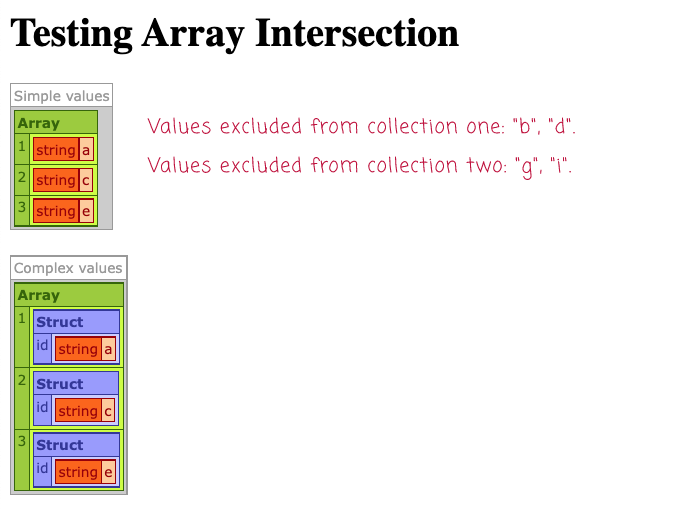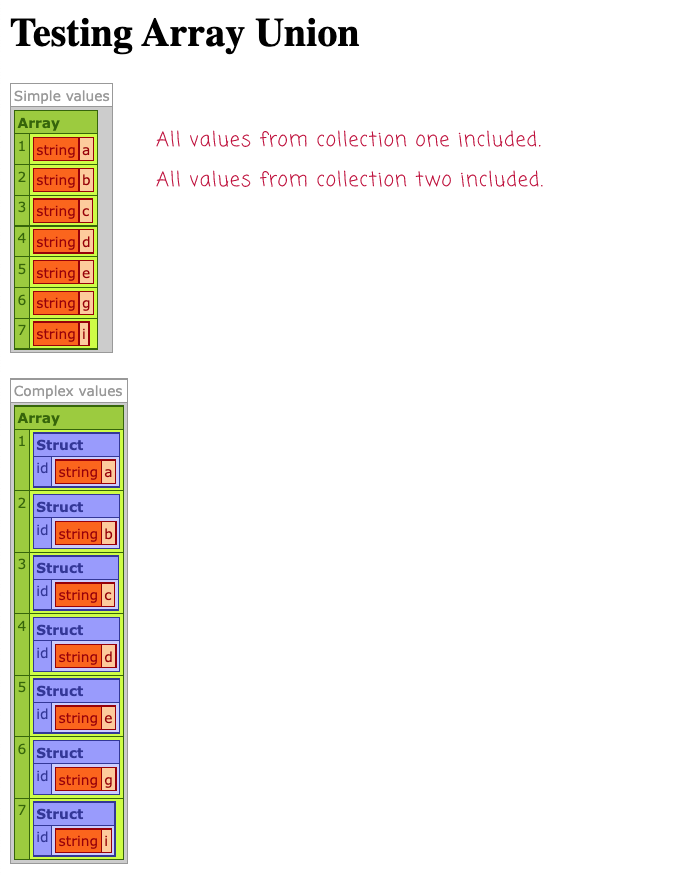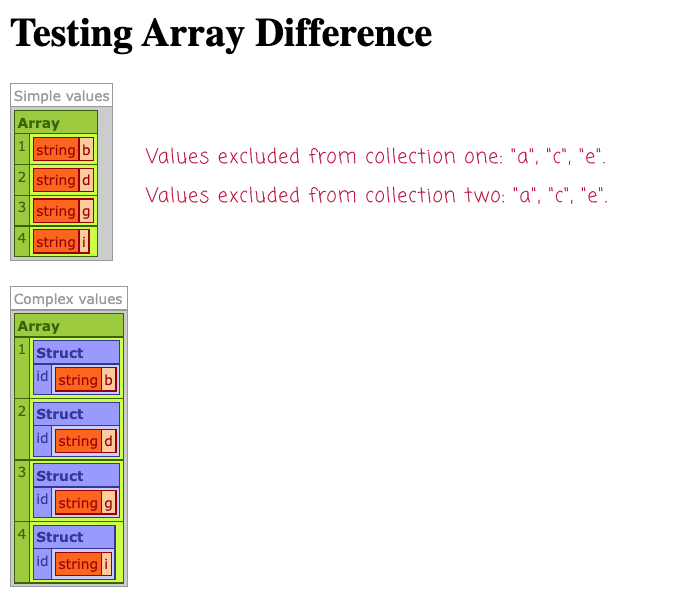# Code Kata: Array Intersection, Union, And Difference In Lucee CFML 5.3.8.201

By on
Tags:

For the last week or so, I've had some extreme tunnel vision at work as I was barrelling towards a deadline to finish building a Mail Blasts feature for InVision. And, now that I'm done (crushing it), I need a little breather. As a fun code kata, I thought I might play around with calculating array interactions, unions, and differences in Lucee CFML 5.3.8.201. This isn't something that comes up often in my day-to-day work. But, it's yet another opportunity to showcase the unyielding power of the Struct as Index pattern in ColdFusion.

Before we look at the code, I think it's important to underscore that this is not an entirely generic solution. My approach makes some assumptions about how I want the collection logic to operate:

• I'm ignoring duplicate entries in all cases - only the first unique item is collected.

• I'm ignoring null / undefined entries in the collection.

• I'm assuming that `3` (number) and `"3"` (string) are the same value. This is a crucial assumption in all of my algorithms because all three functions work by building-up an index internally. And, since all Struct keys in ColdFusion are Strings, `3` (number) and `"3"` (string) both values result in the same Struct key.

• I'm assuming that `A` (uppercase) and `a` (lowercase) are the same value. This is because Struct keys are, by default, case-insensitive. As such, both values result in the same Struct key.

ASIDE: ColdFusion 2021 includes case-sensitive Structs, which would get around this issue. But, it's not something I was worried about.

These are the assumptions that work for me, your mileage may vary. With these assumptions, I am able to create algorithms that require relatively simple logic and can be accomplished with only a single iteration of each collection. Which, I think means this is `O(n)` performance? It's been decades since I've thought about Big-O notation.

All three of my ColdFusion functions allow an optional Closure to be passed-in as the function that maps a given value to its Struct-key. This allows us to compare complex objects in a language that doesn't naturally allow of complex object comparisons.

And, finally, all three of my ColdFusion functions use an Ordered Struct to collect the results. This allows the calculated collection to be generated from the index while maintaining the order in which the values were inspected. I mean, Structs are just kind of an amazing data structure!

With that said, let's get to the algorithms.

## Array Intersection in ColdFusion

With an intersection, we want to find the values that exist in both collections.

``````<cfscript>

echo( "<h1> Testing Array Intersection </h1>" );

// Let's try with simple values.
c1 = [ "a", "a", "b", "c", "d", "e", nullValue() ];
c2 = [ "a", "a", "c", "e", "g", "i", nullValue() ];

dump(
label = "Simple values",
var = arrayIntersection( c1, c2 )
);

// Let's try with complex values that require and identity closure.
c1 = [ { id: "a" }, { id: "a" }, { id: "b" }, { id: "c" }, { id: "d" }, { id: "e" }, nullValue() ];
c2 = [ { id: "a" }, { id: "a" }, { id: "c" }, { id: "e" }, { id: "g" }, { id: "i" }, nullValue() ];

dump(
label = "Complex values",
var = arrayIntersection( c1, c2, ( item ) => item.id )
);

// ------------------------------------------------------------------------------- //
// ------------------------------------------------------------------------------- //

/**
* I return the intersection of the two collections.
*
* NOTE: Internally, the algorithm creates an INDEX of simple values. As such, each
* item needs to either be a simple value; or, you need to pass-in a Closure that can
* generate a simple-value representation of each item. The common items will be
* gathered from the first collection. Any "duplicate" items are ignored.
*/
public array function arrayIntersection(
required array collectionOne,
required array collectionTwo,
function identity
) {

// The algorithm works by iterating over the first collection once and generating
// an index. Then, it loops over the second collection and gathers any value that
// CAN BE MATCHED in the index. As such, we only need to perform 2 loops in order
// to locate the COMMON values.
var index = {};
// NOTE: We're using an ordered struct for the intersection index so that the
// subsequent call to gather the struct-values will return the values in the same
// order in which they were matched.
var intersectionIndex = [:];

// If no closure was passed-in, let's create one that does nothing but return the
// first argument it was given. This way, the rest of our algorithm can operate as
// if a closure was always provided.
identity = ( identity ?: ( value ) => value );

// LOOP ONE: Build the index from the first collection.
for ( var item in collectionOne ) {

// Skip over null / undefined items.
if ( isNull( item ) ) {

continue;

}

var key = identity( item );

// Skip over items that we've already indexed (ie, duplicate items).
if ( index.keyExists( key ) ) {

continue;

}

index[ key ] = item;

}

// LOOP TWO: Gather the COMMON items from the index.
for ( var item in collectionTwo ) {

// Skip over null / undefined items.
if ( isNull( item ) ) {

continue;

}

var key = identity( item );

// Skip over items that we've already collected in the intersection.
if ( intersectionIndex.keyExists( key ) ) {

continue;

}

// If the key exists in the first collection's index, then it exists in both
// collections and should be part of the intersection.
if ( index.keyExists( key ) ) {

intersectionIndex[ key ] = index[ key ];

}

}

return( intersectionIndex.valueArray() );

}

</cfscript>
``````

When we run `arrayIntersection()` in ColdFusion, we get the following output:## Array Union in ColdFusion

With a union, we want to find the values that exist in either collections.

``````<cfscript>

echo( "<h1> Testing Array Union </h1>" );

// Let's try with simple values.
c1 = [ "a", "a", "b", "c", "d", "e", nullValue() ];
c2 = [ "a", "a", "c", "e", "g", "i", nullValue() ];

dump(
label = "Simple values",
var = arrayUnion( c1, c2 )
);

// Let's try with complex values that require and identity closure.
c1 = [ { id: "a" }, { id: "a" }, { id: "b" }, { id: "c" }, { id: "d" }, { id: "e" }, nullValue() ];
c2 = [ { id: "a" }, { id: "a" }, { id: "c" }, { id: "e" }, { id: "g" }, { id: "i" }, nullValue() ];

dump(
label = "Complex values",
var = arrayUnion( c1, c2, ( item ) => item.id )
);

// ------------------------------------------------------------------------------- //
// ------------------------------------------------------------------------------- //

/**
* I return the union of the two collections.
*
* NOTE: Internally, the algorithm creates an INDEX of simple values. As such, each
* item needs to either be a simple value; or, you need to pass-in a Closure that can
* generate a simple-value representation of each item. All unique items from the fist
* collection are included; then, unique items from the second collection are added.
* Any "duplicate" items are ignored.
*/
public array function arrayUnion(
required array collectionOne,
required array collectionTwo,
function identity
) {

// The algorithm works by looping over the first collection and adding all unique
// items to the index. Then, it loops over the second collection and adds any
// items that cannot be found in the index. As such, we only need to perform 2
// loops in order to locate the entire set of values.
// --
// NOTE: We're using an ordered struct for the index so that the subsequent call
// to gather the struct-values will return the values in the same order in which
// they were matched.
var unionIndex = [:];

// If no closure was passed-in, let's create one that does nothing but return the
// first argument it was given. This way, the rest of our algorithm can operate as
// if a closure was always provided.
identity = ( identity ?: ( value ) => value );

// LOOP ONE: Build the index from the first collection.
for ( var item in collectionOne ) {

// Skip over null / undefined items.
if ( isNull( item ) ) {

continue;

}

var key = identity( item );

// Skip over items that we've already indexed (ie, duplicate items).
if ( unionIndex.keyExists( key ) ) {

continue;

}

unionIndex[ key ] = item;

}

// LOOP TWO: Gather the UNCOMMON items from the second collection.
for ( var item in collectionTwo ) {

// Skip over null / undefined items.
if ( isNull( item ) ) {

continue;

}

var key = identity( item );

// Skip over items that we've already collected in the union.
if ( unionIndex.keyExists( key ) ) {

continue;

}

unionIndex[ key ] = item;

}

return( unionIndex.valueArray() );

}

</cfscript>
``````

When we run `arrayUnion()` in ColdFusion, we get the following output:## Array Difference in ColdFusion

With a difference, we want to find the values that exist in one collection but not the other collection.

``````<cfscript>

echo( "<h1> Testing Array Difference </h1>" );

// Let's try with simple values.
c1 = [ "a", "a", "b", "c", "d", "e", nullValue() ];
c2 = [ "a", "a", "c", "e", "g", "i", nullValue() ];

dump(
label = "Simple values",
var = arrayDifference( c1, c2 )
);

// Let's try with complex values that require and identity closure.
c1 = [ { id: "a" }, { id: "a" }, { id: "b" }, { id: "c" }, { id: "d" }, { id: "e" }, nullValue() ];
c2 = [ { id: "a" }, { id: "a" }, { id: "c" }, { id: "e" }, { id: "g" }, { id: "i" }, nullValue() ];

dump(
label = "Complex values",
var = arrayDifference( c1, c2, ( item ) => item.id )
);

// ------------------------------------------------------------------------------- //
// ------------------------------------------------------------------------------- //

/**
* I return the difference of the two collections.
*
* NOTE: Internally, the algorithm creates an INDEX of simple values. As such, each
* item needs to either be a simple value; or, you need to pass-in a Closure that can
* generate a simple-value representation of each item. Any "duplicate" items are
* ignored.
*/
public array function arrayDifference(
required array collectionOne,
required array collectionTwo,
function identity
) {

// The algorithm works by iterating over the first collection once, gathering the
// items, and generating an index. Then, it loops over the second collection and
// gathers any value that CANNOT BE MATCHED in the first index. As such, we only
// need to perform 2 loops in order to locate the UNCOMMON values.
var indexOne = {};
var indexTwo = {};
// NOTE: We're using an ordered struct for the difference index so that the
// subsequent call to gather the struct-values will return the values in the same
// order in which they were matched.
var differenceIndex = [:];

// If no closure was passed-in, let's create one that does nothing but return the
// first argument it was given. This way, the rest of our algorithm can operate as
// if a closure was always provided.
identity = ( identity ?: ( value ) => value );

// LOOP ONE: Build the index from the first collection and gather all items.
for ( var item in collectionOne ) {

// Skip over null / undefined items.
if ( isNull( item ) ) {

continue;

}

var key = identity( item );

// Skip over items that we've already indexed (ie, duplicate items).
if ( indexOne.keyExists( key ) ) {

continue;

}

// The second collection may be empty. As such, let's start off by assuming
// that every item in the first collection might be part of our difference.
indexOne[ key ] = differenceIndex[ key ] = item;

}

// LOOP TWO: Gather the UNCOMMON items.
for ( var item in collectionTwo ) {

// Skip over null / undefined items.
if ( isNull( item ) ) {

continue;

}

var key = identity( item );

// Skip over items that we've already indexed (ie, duplicate items).
if ( indexTwo.keyExists( key ) ) {

continue;

}

indexTwo[ key ] = differenceIndex[ key ] = item;

// If the collected item from the second collection also exists in the first
// collection, remove it from the difference.
if ( indexOne.keyExists( key ) ) {

differenceIndex.delete( key );

}

}

return( differenceIndex.valueArray() );

}

</cfscript>
``````

When we run `arrayDifference()` in ColdFusion, we get the following output:Again, my algorithms make assumptions that allow the logic to remain relatively simple. You could certainly make the algorithms more robust. You could even make them use the triple-equals (`===`) operator for complex object memory comparisons in Lucee CFML. But, all of that would just be edge-case handling (in my opinion). That's why algorithms like this - in a language like ColdFusion which is very flexible - are probably ones you want to write yourself for a specific application. That way, you can bake-in all the assumptions you want!

If nothing else, let's all just observe how amazing the Struct data-type is. As much as Lists are the unsung heroes of ColdFusion, Structs are very much the loudly sung heroes of ColdFusion! Not a day goes by where I don't use a Struct in a fun and exciting way to get the job done.

Want to use code from this post? Check out the license.